# It’s Spring Time Part 4: Energy Absorption, Natural Frequency, and The Surging Phenomenon

In our last post on the spring series, we examine energy absorption, natural frequency, and the surging phenomenon.

When you apply a load on the spring, deflection (deformation) occurs in accordance with Hooke’s law. (See [Fig.1].) When you quickly release a load from this condition, the spring will be restored to its original condition by the oscillating motion. This means that energy by deflection is accumulated in the spring while the load is being applied.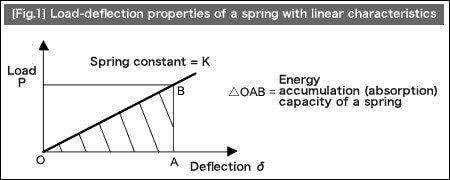The amount of energy accumulated in this spring can be expressed in the following formula:

 Energy accumulated in the spring U = k · δ2 / 2    k: Spring constant  δ: Deflection

This formula is equivalent to the area of the triangle OAB shown in [Fig.1].

If the area of this triangle OAB in [Fig.1] is the energy storage capacity of a spring, the following is true:

 * When the deflection increases, the energy accumulation increases for the same springs. * For different springs, if you increase the amount of deflection, it results in a substantial amount of energy storage capacity even when the spring constant is small.

This principle is applied to shock-absorbing dampers for precision equipment and MechaLock ([Fig.2]).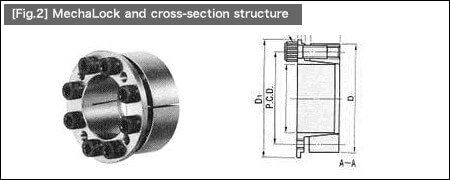Absorbed and Accumulated Energy

Some of the spring structures absorb and accumulate the energy generated during spring deflection.

A ring spring ([Fig.3]) has a structure consisting of inner rings and outer rings with a conical surface alternately stacked. When a pressure load is applied in the axial direction, the outer rings expand and the inner rings contract. Friction then occurs on the conical surface of inner and outer rings. Since this friction causes a part of the deflection energy to be absorbed, ring springs are utilized for shock absorbers and buffer equipment. (See [Fig.4].)
In the case of [Fig.4], the absorbed energy at a release of the deflection is equal to the area enclosed within the load-deflection curve shown in [Fig.4].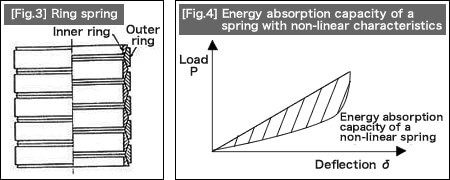Natural Frequency of Springs

When you release the load of this block that was pressing the spring toward the direction shown in [Fig.1], the energy storage property of the spring causes the block to continue oscillating in a vertical direction.Oscillation frequency at this time is the natural frequency (f0) calculated using the following formula: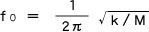The term “Natural frequency” refers to oscillation properties specific to a spring, indicating how many times the vibration is repeated within a certain amount of time.

The value of natural frequency is determined by the two variables: the mass (M) of a load placed and the spring constant (k). Therefore, even when you stretch out the spring or when you slightly pull the spring before releasing, the natural frequency stays the same.

[Fig.2] explains the effect of the mass (A), the effect of the spring constant (B), and the effect of vibration frequency (C), using actual examples.Here are some examples utilizing this spring’s natural frequency as effective properties.

Case examples effectively utilizing the spring’s natural frequency

 Pressing machine } Vibration-proof design and vibration-proofing material Automobile
[Example] Use with a damper to absorb shock from the device.

E.g. Semiconductor equipment —– vibration-proof design and vibration-proofing material

[Example] According to the vibration frequency transmitted from the surrounding area, anti-vibration measures, use of vibration-proof rubber, coil springs, and air suspension are incorporated, as appropriate, into semiconductor equipment (such as precision positioning equipment) that is susceptible to even subtle vibration.

The term “Vibration isolation” (vibration control, vibration absorption) refers to a measure preventing oscillatory propagation to the surrounding area by supporting the vibration-generating equipment with vibration-proofing material.

Vibration removal refers to a measure taken for equipment to be protected from the effect of vibration transmitted from the outside.

Choose a spring whose natural frequency (f0) does not correspond with that of the entire machine (f). (The entire machine is viewed as the spring system here.) Otherwise, the machine needs to be designed to have a different natural frequency from the spring’s frequency. When the spring’s natural frequency (f0) corresponds with that of the entire machine (f), it causes a resonance, maximizing the machine vibration that may trigger accidents, including equipment damage as chatter marks or fracture.

The Surging Phenomenon

When a coil spring absorbs shock, the coil wire undergoes torsion, which is transmitted as a shock wave. This shockwave is called a surge wave.

The amount of time (T) in which this surge wave moves along the spring wire and travels back is called surge time. The surge time and speed can be calculated using the following formula: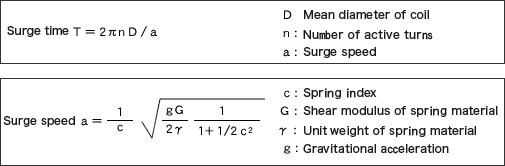When a coil spring is subject to forced oscillation, the resonance phenomenon surging occurs if the cycle corresponds to the surge time T or becomes the half or one-third of the surge time T.

How To Prevent Surging

Changing the cam shape
• Change the cam design to minimize the amplitude of vibration generating from the spring’s natural frequency and cam’s rotation speed in the resonant frequency range.

• Variable pitch springs have the characteristic of a change in deflection altering their natural frequency. Surging can be avoided by adopting a variable pitch spring that does not cause resonance.

Thus this concludes the series on springs. We hope you have picked up a trick or two. Be sure to catch up on the other series!

## The Anatomy of a Micrometer and How to Read Them

A micrometer is one of the instruments used ...